By going through these CBSE Class 11 Chemistry Notes Chapter 4 Chemical Bonding and Molecular Structure, students can recall all the concepts quickly.

## Chemical Bonding and Molecular Structure Notes Class 11 Chemistry Chapter 4

Chemical Bond: The attractive force which holds various constituents (atoms, ions, etc.) together in different chemical species is called.a chemical bond.

There are various theories to explain the formation of a chemical bond. They are

1. Kossel-Lewis approach.
2. Valence-Shell Electron-Pair Repulsion (VSEPR) Theory
3. Valence Bond (VB) Theory
4. Molecular Orbital Theory.

Kossel-Lewis Approach to Chemical Bonding: All noble gases [except He] have 8 electrons in their valence shells. They are chemically inactive. In the case of all other elements, there are less than 3 electrons in the valence shells of their atoms, and hence they are chemically reactive.

“The atoms of different elements combine with each other in order to complete their respective octets (i.e8 electrons in their outermost shell) or duplet (i.e., the outermost shell having 2 electrons in case of H, Li, and Be) to attain stable inert gas configuration”.

Lewis Symbols: Writing symbols of elements with valence shell electrons represented by dots are called Lewis Symbols.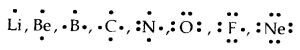→ Significance: The number1 of valence electrons helps to calculate the common or Group Valence of the element. The group valence of the elements is generally either equal to the number of dots in Lewis Symbols or 8 minus the number of dots or valence electrons.

Facts for chemical bonding (Kossel)

1. The highly electronegative halogens and highly electropositive alkali metals are separated by noble gases in the periodic table.
2. The formation of a negative ion and a positive ion is due to the gain or loss of electrons by atoms.
3. The negative and positive ions thus formed attain the nearest noble gas configuration. The noble gases (with the exception of He which has a duplet of electrons) have a particularly stable outer shell configuration of eight (Octet) electrons, ns2np6.
4. The negative and positive ions are stabilized by electrostatic attraction.

Electrovalent or Ionic Bond: When a bond is formed by complete transference of electrons from one atom to another so as to complete their octets or duplets the bond is called an ionic or electrovalent bond. For example→ Formation of Calcium chloride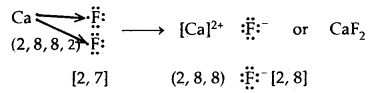→ Formation of MgOThe no. of electrons gained or lost during the formation of an ionic bond is called the electro valency of the elements.

Factors favoring the formation of an ionic bond.

1. Atom going to lose electrons must have low ionization enthalpy so that it can lose electrons readily. Elements of I and II. groups prefer to form, ionic bonds because they have low values of Ionisation enthalpy.
2. Elements that accept electrons must have a High Negative Value of electron gain enthalpy so that they can retain the electrons. Halogens have high values of E.G. enthalpy.
3. Lattice enthalpy should be high. This, in turn, depends upon.
(a) Charge on the ion: Greater the charge on the ion, greater the lattice energy.
(b) Size of the ions: Smaller the size of the ion, the greater the lattice energy.

→ Octet Rule: According to the electronic theory of chemical bonding as developed by Kossel and Lewis, atoms can combine either by transfer of valence electron’s from one atom to another (gain or loss) or by sharing valence electrons in order to have an octet in their valence shells. This is known as the Octet rule.

→ Covalent Bond: A covalent bond is formed as a result of the mutual sharing of electrons between the two atoms to complete their octet or duplet. No. of electrons contributed by each atom is called covalency.

If one pair of electrons (one electron from each atom) is mutually shared between the two atoms, a single covalent bond is formed.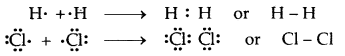If two pairs of electrons (two electrons from each atom) are mutually shared between the two atoms, a double covalent bond is formed.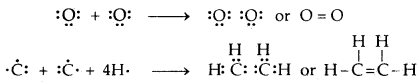If three pairs of electrons (three electrons from each atom) are mutually shared between the two atoms.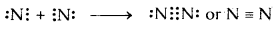Lewis Representation of Simple Molecules (the Lewis Structures)
The Lewis dot structures provide a picture of bonding in molecules and ions in terms of the shared pairs of electrons and the octet rule. While such a picture may not explain the bonding and behavior of a molecule completely, it does help in understanding the formation and properties of the molecules to a large extent. Writing of Lewis dot structure m molecules is, therefore, very useful.

The Lewis-dot-structures can be written by adopting the following steps:
1. The total number of electrons required for writing the
structures is obtained by adding the valence electrons of the combining atoms. For example, in the CH4 molecule, there are eight valence electrons available .for bonding (4 from carbon and 4 from the four hydrogen atoms).

2. For anions, each negative charge would mean the addition of one electron. For cations, each positive charge would result in the subtraction of one electron from the total number of valence electrons. For example, for the CO32- ion, the 2- charges indicate that there are two additional electrons than those provided by the neutral atoms. For NH4+ ion, 1+ charge indicates the loss of the electrons from the group of neutral atoms.

3. Knowing the chemical symbols of the combining atoms and
having knowledge of the skeletal structure of the compound (known or guessed intelligently), it is easy to distribute the total number of electrons as bonding shared pairs between the atoms in proportion to the total bonds.

4. In general the least electronegative atom occupies the central position in the molecule/ion. For example in the NF3 and CO32-, nitrogen and carbon are the central atoms whereas fluorine and oxygen occupy the terminal positions.

5. After accounting for the shared pairs of electrons for single bonds, the remaining electron pairs are either utilized for multiple bonding or remain as the lone pairs. The basic requirement being that each bonded atom gets an octet of electrons.

→ Lewis representation of a few molecules/ions are given in the table below:

Table: The Lewis Representation of Some MoleculesEach H atom attains the configuration of helium (a duplet of electrons)

→ Formal Charge: Lewis dot structures, generally, do not represent the actual shape of the molecules.

The formal charge of an atom in a polyatomic molecule or ion is the difference between the number of valence electrons in that atom in an isolated state or free State and the no. of electrons assigned to that atom in the Lewis structure.

Formal charge (F.C) on an atom in a Lewis structure = [total no. of valence electrons in the free atom] – [total no. of non-bonding or lone pair of electrons] – $$\frac{1}{2}$$ [total no. of bonding (shared) electrons]

The molecule of O3 can be considered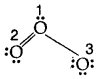The atoms have been numbered 1. 2 and 3

The formal charge on

1. the central atom O marked 1
= 6 – 2 – $$\frac{1}{2}$$  = + 1
2. the end O atom marked 2 = 6 – 4 – $$\frac{1}{2}$$ = 0
3. the end O atom marked 3 = 6 – 6 – $$\frac{1}{2}$$(2) = – 1

Hence we represent O3 along with formal charges as follows:Generally, the lowest energy structure is the one with the smallest formal charges on the atoms. The formal charge is a factor based on a pure covalent view of bonding in which electron pairs are shared equally by neighboring atoms.

Limitations of the Octet Rule: It is mainly useful to understand the structures of organic compounds and applies to the elements of the second period of the periodic table. But it is not universal.

1. The incomplete octet of the central atom: In some compounds, the number of electrons surrounding the central atom is less than eight. This especially is the case with elements having less than four valence electrons. Examples are LiCl, BeH2 and BCl3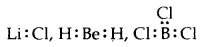Li, Be and B have 1, 2, and 3 valence electrons only. Other such compounds are AlCl3 and BF3.

2. Odd electrons molecules: In molecules with an odd number of electrons like nitric oxide, NO, and nitrogen oxide, NO2, the octet rule is not satisfied for all the atoms.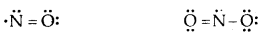3. The expanded octet Elements in and beyond the third period of the periodic table have, apart from 3s and 3p orbitals, 3d orbitals are available for bonding. In a number of compounds of these elements, there are more than eight valence electrons around the central atom. This is termed the expanded octet. The octet rule does not apply in such cases.

Some of the examples of such compounds and PF3, SF6, H2SO4, and a number of coordination compounds.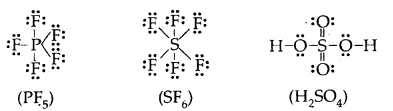4. It is clear that the octet rule is based upon the chemical inertness of noble gases. However, some noble gases (Xenon and Krypton) also combine with oxygen and fluorine to form a number of compounds like XeF2, XeF4, SeOF2, KIF2, etc.

5. The theory does not account for the shape of molecules.

6. It does not explain the relative stability of the molecules being totally silent about the energy of a molecule.

Ionic or Electrovalent Bond: An ionic (or electrovalent) bond is formed by a complete transfer of one or more electrons from the atom of metal to that of a non-metal.

As a result of this electron transfer, the following changes occur in the reacting atoms.
(a) Both the atoms acquire stable noble gas configuration.
(b) The atom that loses its electrons becomes positively charged ions called a cation, whereas the atoms which gain these electrons becomes negatively charged ion called an anion.
(c) The two oppositely charged ions, i.e., ‘he cation and the anion, are then held together by the Coulomb forces of attraction to form an ionic bond.

Thus, an ionic bond that may be defined as the Coulomb force of attraction which holds the oppositely charged ions together is called an ionic bond.
1. Lattice Enthalpy (Lattice energy): The Lattice enthalpy of an ionic solid is defined as the energy required to completely separate one mole of a solid ionic compound into gaseous constituent ions. For example, the lattice enthalpy of NaCl is 788 kJ mol-1. This means that 788 kJ of energy is required to separate to an infinite distance 1 mol of solid NaCl into 1 mol of Na+ (g) and mole of Cl (g).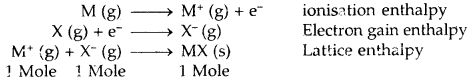For two atoms to form an ionic bond, the following factors are necessary

1. Low ionization enthalpy. The atom going to lose electrons must have a low value of ionization enthalpy.
2. High electron gain enthalpy. The atom going to accept electrons must have a high negative value of electron gain enthalpy.
3. High lattice enthalpy. The lattice energy of the compound should be high. The greater the charge on the ion and the smaller the size, the greater is the lattice enthalpy.

An ionic bond is formed if lattice energy and electron affinity took together are greater than ionization energy. The larger the negative value of lattice energy greater is than the stability of the ionic compound. The stability of an ionic compound is provided by its enthalpy of lattice formation and not simply by achieving an octet of electrons around the ionic species in a gaseous state.

Example of Ionic BondOne Na+ is surrounded by C Cl ions and vice-versa Rock Salt Structure
1. Na+ Cl (Ionic Solid)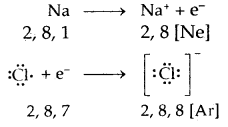2. MgO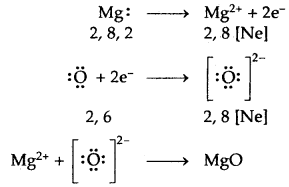3. CaF2Properties of Ionic solids:

1. Ionic compounds are solids, whereas covalent compounds can be solids, liquids, or gases.
2. Ionic compounds do not contain molecules but are made up of ions, whereas covalent compounds are molecular.
3. Ionic compounds have high melting points and boiling points due to strong electrostatic forces of attraction between ions. Covalent compounds, on the other hand, have low melting points and boiling points.
4. Ionic compounds are generally soluble in water or any polar
solvent whereas covalent compounds are insoluble in water, but soluble in non-polar solvents.
5. Ionic compounds take part in ionic reactions whereas covalent compounds take part in molecular reactions.
6. Ionic bonds are non-directional in nature, whereas covalent bonds have directional characteristics.

Bond Parameters
1. Bond length: Bond length is defined as the equilibrium distance between the nuclei of two bonded atoms in a molecule. Bond lengths are measured by spectroscopic, X-ray diffraction, and electron diffraction techniques. Each atom of the bonded pair contributes to the bond length (Fig.) In the case of a covalent bond, the contribution from each atom is called the covalent radius of that atom.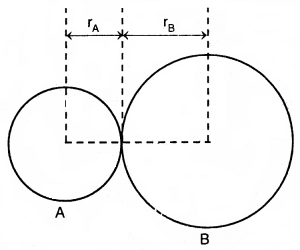The bond length in a covalent molecule AB
R = rA + rB (R is the bond length and rA and rB are the covalent radii of atoms A and B respectively)

The covalent radius is measured approximately as the radius of an atom’s core which is in contact with the core of an adjacent atom in a bonded situation. The covalent radius is half of the distance between two similar atoms joined by a covalent bond in the same molecule.

The van der Waals radius represents the overall size of the atom which includes its valence shell in a nonbonded situation. Further, the van der Waals radius is half of the distance between two similar atoms in separate molecules in a solid. Covalent and van der Waals radii of chlorine and depicted in Fig.Covalent and van der Waals radii in a chlorine molecule. The inner circles correspond to the size of the chlorine atom (rvdw and r0 are van der Waals and covalent radii respectively)

2. Bond angle: It is defined as the angle between the orbitals containing bonding electron pairs around the central atom in a molecule/complexion. For example, the bond angle in water can be represented as under: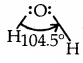3. Bond enthalpy: It is defined as the amount of energy required to break one mole of bonds of a particular type between two atoms in a gaseous state.

The unit of bond enthalpy is kJ mol-1 For example H-H bond enthalpy in hydrogen molecule is 435.8 kJ mol-1
H2 (g) → H (g) + H (g); ΔH = 435.8 kj mol-1

4. Bond order: In the Lewis description of the covalent bond the bond order is given by the number of bonds between the two atoms in a molecule. The bond order in H2 is one, in O2 it is two and in N2 it is three.

Isoelectronic molecules and ions have identical bond orders. For example F2 and O22- have bond order = 1.N2, CO and NO+ have bond order 3.

The stabilities of molecules can be understood by the statement. With the increase in bond order, bond enthalpy increases and bond length decreases.

5. Resonance structures: According to the concept of resonance, whenever a single Lewis structure cannot describe a molecule accurately, a number of structures with similar energy, positions of nuclei, bonding, and non-bonding pairs of electrons are taken as the canonical structures of the resonance hybrid which describes the molecule accurately. Thus for Cl the two structures shown below constitute the canonical structures and their hybrid, (III, below) represents the structure of O, more accurately. Resonance is represented by a double-headed arrow.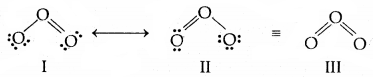Resonance in O3 molecule. I and II represent the two canonical forms III is the resonance hybrid.

Some of the other examples of resonance structures are provided by the carbonate ion and the carbon dioxide molecule.
1. Carbonate ion (CO3)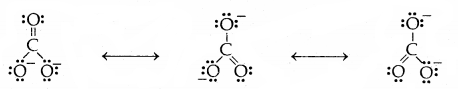2. Carbon-dioxide6. Electronegativity: The electronegativity is the ability of an atom of an element in a molecule to attract electrons towards itself in a shared pair of electrons.

Table: Difference between electronegativity and electron affinity (electron gain enthalpy)

 Electronegativity Electron affinity (electron gain enthalpy) 1. It is the tendency of an atom to attract shared pair of electrons. 1. It is the tendency of an atom to attract outside electrons. 2. It is the property of a bonded atom. 2. It is the property of an isolated atom. 3. The elements with symmetrical configurations have specific electronegativities. 3. The elements with symmetrical configurations have almost zero electron affinities. 4. It has no units. 4. It has units of kJ mol-1 of V atom-1

7. Polarity of Bonds: An ionic bond is formed due to the complete transfer of electrons from one atom to another. A covalent bond is formed due to the mutual sharing of electrons. A covalent bond between two atoms of different elements is called a polar covalent bond. A polar bond is partly covalent bond and partly ionic. The percentage of ionicity in a covalent bond is called the percentage ionic character in that bond. The ionic character in a bond is expressed in terms of bond dipole moment (μ).

The dipole moment of a bond depends upon the difference in the electronegativity of the two atoms held together by the chemical bond.

The dipole moment of two and opposite charges is given by the product of the charge and the distance separating them. Thus
Dipole moment, μ = charge (q) × Distance separation (r)
= q × r

In the HCl molecule, the chlorine is more electronegative. Therefore, the bonding electrons lie closer to the chlorine atom. As a result, H- atom develops a slight positive (+q) and Cl atom a slight negative (-q) charge, thereby generating a dipole in the HC1 molecule.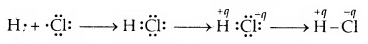For non-polar bonds, there is no charge separation. Therefore, q = 0. As a result, the dipole moment of a non-polar bond is zero.

Dipole moment and the ionic character in bonds: Ionic character in a covalent bond depends upon the difference between the electronegativity of two atoms. The greater the electronegativity difference, the greater is the charge separation, and therefore, the greater is the ionic character in the bond.

The valence shell electron pair repulsion (VSEPR) theory: The main postulates of the VSEPR theory are:

1. Pairs of electrons in the valence shell of a central atom repel each other.
2. These pairs of electrons tend to occupy positions in space that minimize repulsion and thus maximize distances between them.
3. The valence shell is taken as a sphere with the electron pairs localizing on the spherical surface at a maximum distance away from one another.
4. Multiple bonds are treated as if it is a single electron pair and the two or the three electron pairs of multiple bonds are treated as single super pair.
5. Where two or more resonance structures can depict a molecule, the VSEPR model is applicable to any such structure.

Predicting the shape of molecules on the basis of VSEPR theory: According to the VSEPR theory/the geometry of a molecule is determined by the number of electron pairs around the central atom. So to predict the geometry of the molecules, no. of electron pairs (both shared and one pair) should be known.

The repulsive interactions of electron pairs decrease in the order l – l – p > l – pb – p > b – pb – p repulsions.

The geometry of Molecules in which the Central Atom has No Lone Pair of Electrons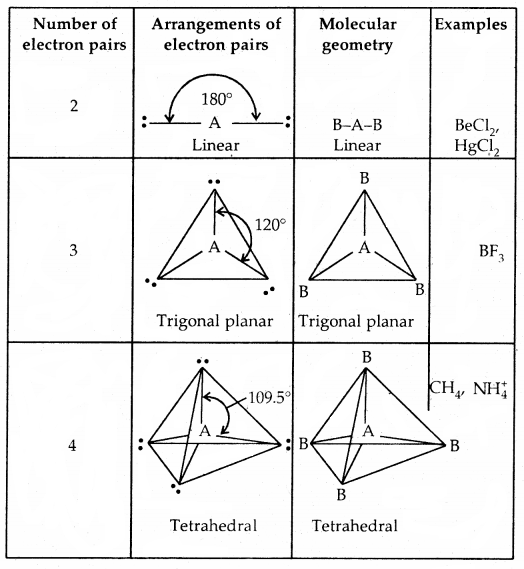Shapes (Geometries) of some simple molecules/ions with central Ions having One or More Lone Pairs of Electron (E)Shapes of Molecules containing Bond Pair and Lone Pair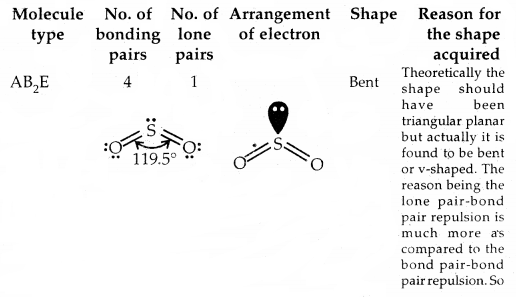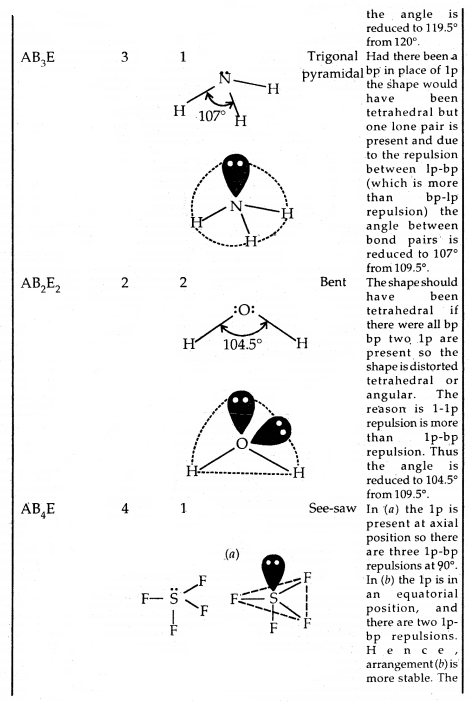Valence Bond Theory: The main postulates of the valence bond theory are:

1. A covalent bond is formed due to the overlap of the outermost half-filled orbitals of the combining atoms. The strength of the bond is determined by the extent of overlap.
2. The two half-filled orbitals involved in the covalent bond formation should contain electrons with opposite spins. The two electrons then move under the influence of both nuclei.
3. The completely-filled orbitals (orbitals containing two paired electrons) do not take part in the bond formation.
4. An s-orbital does not show any preference for direction. The non-spherical orbitals such as p-and d-orbitals tend to form bonds in the direction of the maximum overlap, i.e., along the orbitals axis.
5. Between the two orbitals of the same energy; the orbital which is non-spherical (e.g., p- and d-orbitals forms stronger bonds than the orbitals which are spherically symmetrical, e.g., s-orbital.
6. The valence of an element is equal to the number of half-filled orbitals present in it.

In the valence bond model, the stability of a molecule is explained in terms of the following type of interactions:
(a) electron-nuclei attraction interactions, i.e., the electrons of one atom are attracted by the nucleus of the other atom also.
(b) electron-electron repulsive interactions, i.e., electrons of one atom are repelled by the electrons of the other atom.
(c) nucleus-nucleus repulsive interactions, i.e., the nucleus of one atom is repelled by the nucleus of the other atom.

Valence Bond Description of Hydrogen molecule: A hydrogen molecule is a stable molecule. That is why one mole of H2 molecules requires energy equal to 433 kJ to dissociate into hydrogen atoms, viz.
H2 (g) + 433 kJ → 2H (g)

The formation of a hydrogen molecule from two hydrogen atoms may be considered to take place through the following steps:
Step 1. Consider two hydrogen atoms HA and HB at large separation from each other, so that there is no interaction between them. Since, there is no interaction between the two H atoms, hence the total energy is equal to the sum of the energies of the two H atoms. (Fig. a)(a) No interactions at large distances

Step 2. Now, let the two H atoms approach each other. When the two atoms come closer new attractive and repulsive forces begin to operate. The electrons of one atom are attracted by the nucleus of the other atom. At this stage, both the electrons are attracted by both nuclei. Since, attractive interactions lead to a decrease of energy, hence as the two(b) Interactions start as atoms come closer.
atoms approach each other the energy of the system starts decreasing. (Fig. b, e, d).

Step 3. When two hydrogen atoms, HA and HB come still closer the electron-electron (eA — eB) and the nucleus- nucleus repulsive interactions start operating. The repulsive interaction tends to increase energy. As long as the attractive interactions are stronger than the repulsive interactions, the energy of the system continues to decrease.

At a certain distance between the two atoms, the attractive and repulsive interaction balance each other, and the energy of the system attains a minimum value. At the state, two H atoms have a fixed distance between them and form a stable H2 molecule. The internuclear separation when the energy of the system is minimum is called bond length The H—H bond length in H2(g) is 75 pm (0.74 A).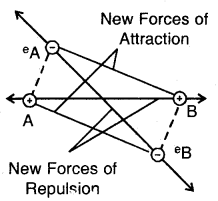New forces of attraction and repulsion between two H-atoms approaching each other

Step 4. Now, if the two H- atoms in an H2 molecule are formed to come closer than the equilibrium internuclear separation (bond length: 74 pm), the repulsive forces start predominating and as a result, the energy of the system increases very sharply (Fig. above)(d) Potential energy diagram showing the variation of energy with the internuclear distance between two H-atoms

2. Overlap of atomic orbitals: Various types of atomic orbitals overlap leading to the formation of covalent bonds are:
(a) s-s overlap: In this type of overlap, half-filled s-orbital of the two combining atoms overlap each other.(b) s-p overlap: Here a half-filled s-orbital of one atom overlaps with one of the p-orbitals having only one electron in it.

(c) p-p overlap along the orbital axis: This is called head-on, end-on, or end-to-end linear overlap. Here, the overlap of the two half-filled p-orbitals takes place along the line joining the two nuclei.(d) p-p sideways overlap: This is also called lateral overlap, in this type of overlap, two p-orbitals overlap each other along a line perpendicular to the internuclear axis, i.e., the two overlapping p- orbitals are parallel to each other.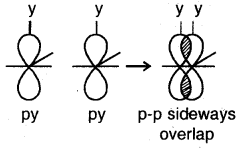The p-p sideways overlap

Hybridization of Atomic Orbitals:
Hybridization: It may be defined as the phenomenon of mixing of orbitals of nearly the same energy so as to redistribute their energies and to give rise to new orbitals of equivalent energies and shapes.

The new orbitals that are formed are called hybridized or hybrid orbitals. As a general rule, the number of hybrid orbitals produced from hybridization is equal to the number of orbitals that are mixed together. For example, when an s orbital is mixed with a p orbital two sp hybrid orbitals are produced. The two orbitals lie in a straight line and make an angle of 180°. Examples of molecules with sp hybrid orbitals are BeF2 and CH ≡ CH (acetylene).The ground state configuration of the boron is 1 s22s22p1x. If one of the 2s electrons is promoted to an orbital of a little higher energy, say 2p1y orbital, then the configuration 1s2 2s1 2p1 2p1y would result. The three equivalent orbitals formed from one 2s and two 2p orbitals are called sp2 hybrid orbitals, which are coplanar and directed at an angle of 120° to each other as shown. The orbitals form a stronger bond than the sp orbitals.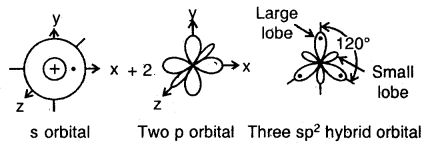sp3 Hybridization: The ground state configuration of carbon = 6 = 1s2, 2s2, 2px1 2p1y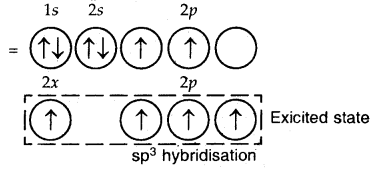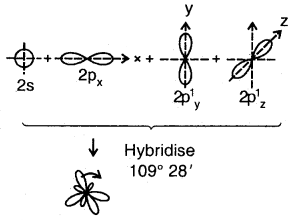4sp3 hybrid orbitals of C

The four sp3 hybrid orbitals are directed towards the four corners of the tetrahedron. The bond angles are 109°28′.

Example of sp3 hybridization: Shape of CH4
The shape of CH4 Molecule4sp3 hybrid orbitais of C

Sigma (σ) and Pi (π) bonds
1. Sigma (σ) Bond: It is formed as a result of s-s, s-p, or p-p overlap axially or on the internuclear axis. A sigma bond îs strong as a result of overlapping on the internuclear axis.
1. Combination of s-orbitais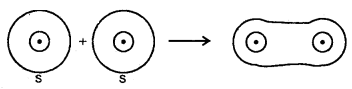2. Combina Lion of s- and p- orbitais bond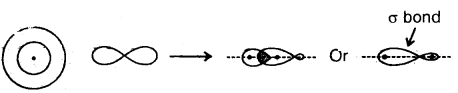3. End to end combination of two p-orbitals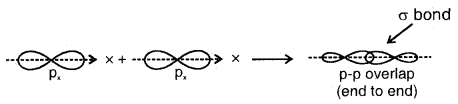2. Pi (π) Bond: When a bond is formed by the lateral or sideways overlapping of atomic orbitals, it is known as a pi (π) bond. A π bond is weaker than a bond as the overlapping is not effective and is made up of two half-electron-clouds, 1/2 above and 1/2 below the internuclear axis. It is always present in addition to ao bond in molecules containing a double bond or a triple bond. Between two atoms forming a single covalent bond, it has to be a bond. A Pi (π) bond has no primary effect on the direction of the bond. It however shortens the internuclear distance.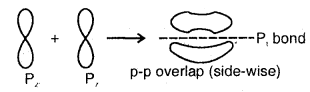The relative bond strength.has the order p-p < s-p < s-s

In addition to the sp hybridization in BeF2 sp2 hybridization in BF3 and sp3 hybridization in CH4, we have hybridization involving d-orbitals also.

The elements of the third period contain d-orbitals also in addition to s and p orbitals. The 3d orbitals are comparable in energy to 3s and 3p orbitals.

Due to the availability of d orbitals, valencies of 5, 6, and 7 are also expected.
1. sp3d hybridization. This involves the mixing of one s three p and one d-orbitals. These orbitals hybridize to form five sp3d hybrid orbitals which are directed towards the corners of a regular trigonal bipyramidal geometry. For example, PF5 has this geometry.

2. sp3d2 hybridization. This involves the mixing of one-s orbital, three p, and two d-orbitals. These six orbitals hybridize to form, six sp3d2 hybrid orbitals which are directed towards the corners of an octahedral geometry. For example, the SF6 molecule has octahedral geometry.

3. sp3d3 hybridization. This involves the mixing of one s, three p, and three-d orbitals forming seven sp3d3 hybrid orbitals. These adopt pentagonal bipyramidal geometry. For example, IF7 has this geometry.

4. dsp2 hybridization. In this case, one d(dx2– y2), one, and two p orbitals get hybridized to form four dsp2 hybrid orbitals. These hybrid orbitals have square planar geometry. For example [Ni(CN)4]2- ion.

Molecular Orbital Theory: Molecular orbital theory was developed by Hund and Milliken in 1932. The basic idea of molecular orbitals theory is that atomic orbitals of individual atoms combine to form molecular orbitals. The electrons in molecules are present in these molecular orbitals which are associated with several nuclei. These molecular orbitals are filled in the same way as the atomic orbitals in atoms are filled.

The molecular orbitals are formed by the combination of atomic orbitals of the bonded atoms.

The salient features of the Molecular orbital theory are:

1. The electrons in a molecule are present in the various molecular orbitals as the electrons of atoms are present in the various atomic orbitals.
2. The atomic orbitals of comparable energies and proper symmetry combine to form molecular orbitals.
3. While an electron in an atomic orbital is influenced by one nucleus, in a molecular orbital, it is influenced by two or more nuclei depending upon the number of atoms in the molecule. Therefore, we can call- a molecule orbital Polycentric.
4. The no. of MOs formed is equal to the no. of combining AOs. When two atomic orbitals combine, two MOs are formed. One is called bonding molecular orbital and the other is called an antibonding molecular orbital.
5. The bonding MO has lower energy and hence greater stability than the corresponding antibonding MO.
6. Just as the electron probability distribution around a nucleus in an atom is given by an atomic orbital the electron probability distribution around a group of nuclei in a molecule is given by a molecular orbital.
7. The MOs obey the Aufbau principle, Pauli Exclusion principle, and Hund’s rule like AOs.

Formation of Molecular Orbitals/Linear Combination of Atomic Orbitals (ICAO)
If φA and φB represent the wave functions of atomic orbitals A and B of hydrogen atoms forming hydrogen molecule, then
φMO = φA ± φB
Therefore the two molecular orbitals a and a* are formed as follows:
σ = φA + φB
σ* = φA – φB
where σ is called bonding molecular orbital and σ* is called an antibonding molecular orbital.

For example, s-orbitals of two hydrogen atoms combine to form two MOs — σ1s and σ*1s (bonding and antibonding MOs).

Similarly, 2s atomic orbitals may combine to form two MOs: σ2s and σ*2s.
If the 2 axes is assumed to be the internuclear axis, then 2p atomic orbitals combine to form sigma orbitals. The 2pz atomic orbitals combine to form aMOs (σ2pz, σ*2pz) while 2px and 2py orbitals combine to form nMOs (π2px, π*2px, π2py, π*2py).

Energy Level Diagrams for Molecular Orbitals: 1s, 2s and 2p orbitals of two atoms combine to form bonding MOs: σ1s, σ2s, σ2pz, π2px, π2py. and antibonding MOs:

σ*l s,’ σ*2s, σ*2pz, π*2px and π*2py. The energy levels of these molecular orbitals have been determined experimentally by spectroscopic methods. It is observed that for diatomic molecules of the second period Li2 to Ne2 there are two types of energy levels of MOs. For molecules Li2, Be2, B2, C2, and N2 the sequence of energies of MOs may be written as
σ1s, σ*ls, σ2s, σ*2s, π2px = π2py, σ2pz, π*2px π*2py, σ*2pz

On the other hand, for the molecule 02, F2 and Ne2.the sequence of energies of MOs are:
σ1s, σ1s, σ2s, σ*2s, σ2pz, π2px = π2py, π*2px = π*2py, σ*2pz.

The main difference between the two types of sequences is that for molecules O2, F2 and Ne2, the σ2pz MO is lower in energy than π2px and π2py MOs while in the case of molecules Li2, Be2, B2, C2, and N2, σ2pz MOs higher energy than π2px arid π2py MOs.

Bond Order: It is defined as the number of covalent bonds in a molecule. Bond order can .be calculated from the number of electrons in bonding and antibonding molecular orbitals as:

Bond order = $$\frac{\text {No. of electrons in bonding MOs-No. of electrons in anit-bonding MOs }}{2}$$
or
= $$\frac{\mathrm{N}_{b}-\mathrm{N}_{a}}{2}$$

The bond orders of 1, 2, or 3 correspond to the single, double, or triple bonds. But bond order may be fractional also in some cases.

Information Conveyed by Bond Order.

1. If the value of bond order is positive; it indicates a stable molecule and if the value of bond order is negative or zero, it means that the molecule is unstable.
2. The stability of a molecule is measured by its bond
dissociation energy. But the bond dissociation energy is directly proportional to the bond order.
3. Bond order is inversely proportional to the bond length. The higher the bond order value, the smaller is the bond length.
4. If all electrons are doubly occupied in different molecular orbitals the molecule is diamagnetic. If there are one or more unpaired electrons, it is paramagnetic like O2.

Conditions for the combination of Atomic Orbitals:

1. The combining atomic orbitals must have the same or nearly the same energy. This means that Is orbital can combine with another 1s, but not with 2s orbitals because the energy of 2s is appreciably higher than that of Is.
2. The combining atomic orbitals must have the same symmetry as the molecular axis.
3. The combining atomic orbitals must overlap to the maximum extent. In this type, sigma (σ) molecular orbitals are symmetrical around the bond axis while pi (π) molecular orbitals are not symmetrical. If the internuclear axis is taken to be in the Z-direction it can be seen that a linear combination of 2pz-orbitals of two atoms also produces two sigma molecular orbitals σ2pz and σ*2pz.

Molecular orbitals obtained from 2px and 2py orbitals are not symmetrical around the bond axis because of the positive lobes above and negative lobes below the molecular plane. Such molecular orbitals are labeled as π and π*. A π bonding MO has a large electron density above and below the internuclear axis. The σ* antibonding MO has a node between the nuclei.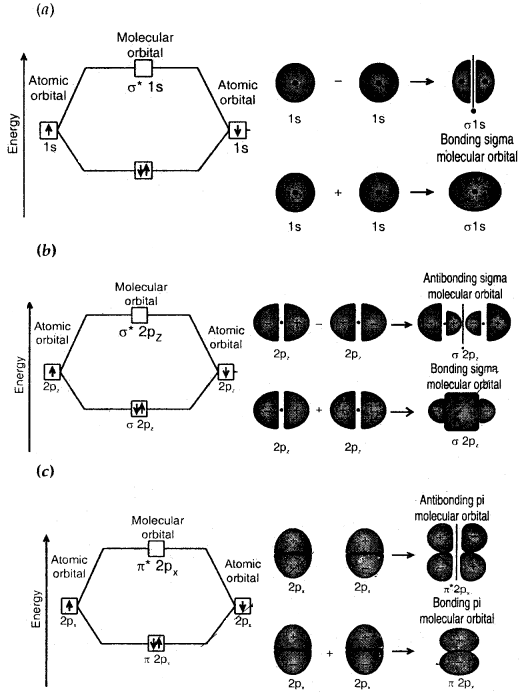Contoursmnd energies of bonding and antibonding molecular orbitals formed through combinations of (a) Is atomic orbitals; (b) 2pz atomic orbitals and (c) 2px atomic orbitals

Molecular Orbital Electronic Configurations: Molecular orbital electronic configurations of some molecules/ions are given below: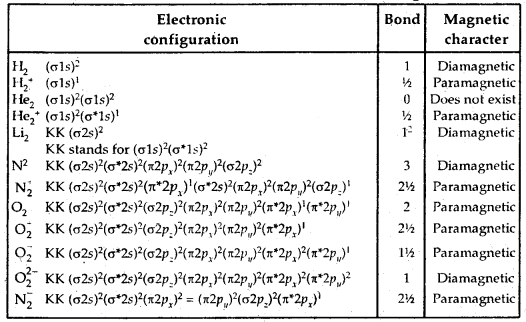→ Hydrogen Bond: The attractive force which binds the hydrogen atom of one molecule with the electronegative atom (F, O, N) of another molecule is known as a hydrogen bond.

A hydrogen bond is weaker than a covalent bond. The strength of a hydrogen bond ranges from 10 – 40 kJ mol-1 while that of a normal covalent bond is of the order of 400 kJ mol-1

Cause of formation of the hydrogen bond. When hydrogen is bonded to a strongly electronegative element, A (such as F, O, or N) the electron pair shared between the two atoms lies far away from the hydrogen atom. As a result, the hydrogen atom becomes highly electropositive with respect to the other atom, A.

Since the electrons are displaced towards A, it acquires partial charge (δ+). In other words, the bond H-A becomes polar and may be represented as Hδ+ – Aδ. The electrostatic force of attraction between positively charged hydrogen- atom of one molecule and negatively charged atom of neighboring molecule results in the formation of the hydrogen bond. This may be represented as:Conditions for Hydrogen Bonding: The conditions for hydrogen bonding are:

1. The molecule must contain a hydrogen atom attached to the highly electronegative atom (F, O, or N).
2. The size of the electronegative atom should be quite small. (HF), water (H2O), ammonia (NH,) alcohols (ROH), carboxylic acids (RCOOH), amines (RNH2), etc. Due to hydrogen bonding, some of the properties of these molecules are influenced.

→ Types of Hydrogen Bonds: Hydrogen bonding may be classified into two types.
(a) Intermolecular hydrogen bond: It is a hydrogen bond formed between two different molecules of the sai^e or different substances. This type of linking results in the association of molecules and increases the melting point, boiling point, viscosity, solubility, etc.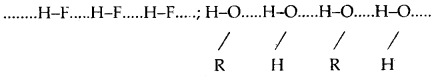(b) Intramolecular hydrogen bond: Intramolecular hydrogen bond is formed between the hydrogen atom and the highly electronegative atom (F, O, or N) present in the same molecule. An intramolecular hydrogen bond results in the cyclization of the molecules and prevents their association. Consequently, the effect of intramolecular hydrogen bonds on physical properties is negligible.

For example, intramolecular hydrogen bonds are present in molecules such as o-nitro phenol, o-nitro benzoic acid, etc.
Ortho nitro phenol Ortho nitrobephobic acid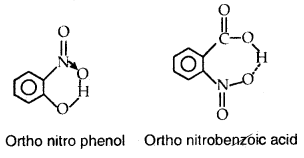→ Chemical Bond: The force which holds the constituent atoms together with a molecule is called a chemical bond.

→ Electrovalent or Ionic bond: A bond formed by the complete transference of electrons from one atom to another to acquire the stable electronic configuration of the nearest gas is called an Ionic bond.

→ Electrovalency: The number of electrons so transferred (gained or lost) is called the electrovalency of the element.

→ Covalent Bond: When two atoms share the electrons mutually in order to complete their octet or duplet, the bond so formed is called a covalent bond.

→ Coordinate Bond: When the electron pair shared between the two atoms is donated by one of the two atoms, it is called a coordinate bond.

→ Sigma (σ) bond: When a bond is formed between the two atoms by the overlap of their atomic orbitals along the internuclear axis (end to end of head-on overlap) the bond so formed is called sigma (σ) bond.
It is due to s-s, s-p, p-p overlap.

→ Pi (π) Bond: It is formed by the side-wise or lateral overlap of only p-orbitals in a direction perpendicular to the internuclear axis.

1. Pi (π) bond is weaker than a sigma (σ) bond.
2. Whenever a Pi (π) bond is formed, it is formed in addition to a sigma (σ) bond in multiple bonds (a double or triple bond)
3. A single bond between the 2 atoms is always a sigma (σ) bond.

→ Bond length: The average distance between the centers of the nuclei of the two bonded atoms is called it’s the bond length.

→ Bond Enthalpy (Bond Energy): The energy required to break one mole of a particular type of bond so as to separate them into gaseous atoms is called bond dissociation energy or bond energy.

→ Dipole Moment: The product of the magnitude of charge (q) and the distance (d) between the centers of charges is called a dipole moment. Mathematically
Dipole moment = µ = q × d

→ Hybridization: It is the phenomenon of mixing of the atomic orbitals belonging to the same atom but having slightly different energies so that redistribution of energy takes place between them resulting in the formation of new orbitals of equal energies and identical shapes.

→ Hydrogen bonding: It can be defined as the attractive force which binds the hydrogen atom of one molecule with the more electronegative atom (like F, O, or N) of another molecule.

1. Intermolecular hydrogen bond: It is formed between two different molecules of the same or different compounds like H-bonds in water (H2O), alcohol (R-OH), and HF.
2. Intramolecular hydrogen bond: It is formed between the H atom and the more electronegative atom (F, O, or N) within the same molecule as in o-nitrophenol.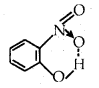→ Ionic compound: It is a three-dimensional aggregation of positive and negative ions in an ordered arrangement called the crystal lattice.

→ Valence Shell Electron Pair Repulsion (VSEPR) Theory: According to this theory used to explain the geometrical shapes of molecules-molecular geometry is determined by repulsions between lone pairs and bond pairs as follows: The order of these repulsions is lone pair-lone pair > lone pair-bond pair > bond pair-bond pair.

→ Bond Angle: It is defined as the angle between the orbitals containing bonding electron pairs around the central atom in a molecule or complexion.The bond angle in the water of H-O-H is 104.5° as shown above.

→ Bond enthalpy: It is defined as the amount of energy required to break one mole of bonds of a particular type between two atoms in a gaseous state. The unit of bond enthalpy is kJ m-1.

→ Bond order: In the Lewis description of covalent bond, the Bond order is given by the number of bonds between the two atoms in a molecule, e.g., bond orders in H2 (H-H), O7(O = O), and N2(N = N) are 1, 2 and 3 respectively.

→ Resonance: Whenever a single Lewis structure cannot explain all the observed properties of a molecule, a number of structures with almost similar energy, positions of the nuclei, bonding, and non-bonding pairs of electrons are taken as the canonical structures of the hybrid which describes the molecule accurately.